# Aligned Induction

## MNIST - Model induction

MNIST - handwritten digits/Model induction

We shall analyse this dataset using the NISTPy repository which depends on the AlignmentRepaPy repository.

### Sections

Model 1 - 15-fud

Model 2 - 15-fud

Model 35 - All pixels

Model 34 - Averaged pixels

Model 5 - 15-fud square regions of 10x10 pixels

Model 6 - Square regions of 10x10 pixels

Model 21 - Square regions of 15x15 pixels

Model 10 - Centred square regions of 11x11 pixels

Model 24 - Two level over 10x10 regions

Model 25 - Two level over 15x15 regions

Model 26 - Two level over centred square regions of 11x11 pixels

NIST test

NIST conditional

Model 36 - Conditional level over 15-fud

Model 37 - Conditional level over all pixels

Model 38 - Conditional level over averaged pixels

NIST test averaged

NIST conditional over square regions

Model 43 - Conditional level over square regions of 15x15 pixels

Model 40 - Conditional level over two level over 10x10 regions

Model 41 - Conditional level over two level over 15x15 regions

Model 42 - Conditional level over two level centred square regions of 11x11 pixels

### Model 1 - 15-fud

Consider a 15-fud induced model of 7500 events of the training sample. We shall run it in the interpreter,

from NISTDev import *

(uu,hrtr) = nistTrainBucketedIO(2)

digit = VarStr("digit")
vv = uvars(uu)
vvl = sset([digit])
vvk = vv - vvl

hr = hrev([i for i in range(hrsize(hrtr)) if i % 8 == 0],hrtr)

(wmax,lmax,xmax,omax,bmax,mmax,umax,pmax,fmax,mult,seed) = (2**10, 8, 2**10, 10, (10*3), 3, 2**8, 1, 15, 1, 5)

(uu1,df) = decomperIO(uu,vvk,hr,wmax,lmax,xmax,omax,bmax,mmax,umax,pmax,fmax,mult,seed)



This runs in Python 3.5 64-bit on a Ubuntu 16.04 Intel(R) Xeon(R) Platinum 8175M CPU @ 2.50GHz in 1419 seconds.

summation(mult,seed,uu1,df,hr)
# (140030.31386191736, 69011.41886741124)

len(dfund(df))
152

len(fvars(dfff(df)))
552

open("NIST_model1.json","w").write(decompFudsPersistentsEncode(decompFudsPersistent(df)))



Imaging the fud decomposition,

pp = treesPaths(hrmult(uu1,df,hr))

rpln([[hrsize(hr) for (_,hr) in ll] for ll in pp])
# [7500, 2584, 1039]
# [7500, 2584, 1543, 812]
# [7500, 4815, 1869, 642]
# [7500, 4815, 1869, 1207, 686]
# [7500, 4815, 2919, 1263, 858]
# [7500, 4815, 2919, 1545, 622]

file = "NIST.bmp"

bmwrite(file,ppbm(uu,vvk,28,2,2,pp))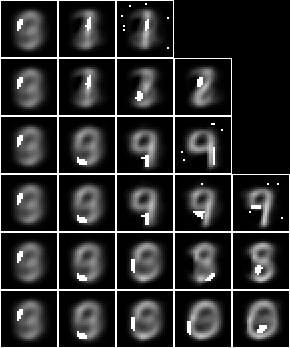The bitmap helper function, ppbm, is defined in NISTDev,

def ppbm(uu,vvk,b,c,d,pp):
kk = []
for ll in pp:
jj = []
for ((_,ff),hrs) in ll:
jj.append(bmborder(1,bmmax(hrbm(b,c,d,hrhrred(hrs,vvk)),0,0,hrbm(b,c,d,qqhr(d,uu,vvk,fund(ff))))))
kk.append(bminsert(bmempty((b*c)+2,((b*c)+2)*max([len(ii) for ii in pp])),0,0,bmhstack(jj)))
return bmvstack(kk)


Both the averaged slice and the fud underlying are shown in each image to indicate where the attention is during decomposition.

### Model 2 - 15-fud

Now consider a similar model but with a larger shuffle multiplier, mult,

from NISTDev import *

(uu,hrtr) = nistTrainBucketedIO(2)

digit = VarStr("digit")
vv = uvars(uu)
vvl = sset([digit])
vvk = vv - vvl

hr = hrev([i for i in range(hrsize(hrtr)) if i % 8 == 0],hrtr)

(wmax,lmax,xmax,omax,bmax,mmax,umax,pmax,fmax,mult,seed) = (2**10, 8, 2**10, 10, (10*3), 3, 2**8, 1, 15, 3, 5)

(uu1,df) = decomperIO(uu,vvk,hr,wmax,lmax,xmax,omax,bmax,mmax,umax,pmax,fmax,mult,seed)



This runs in Python 3.5 64-bit on a Ubuntu 16.04 Intel(R) Xeon(R) Platinum 8175M CPU @ 2.50GHz in 1507 seconds.

summation(mult,seed,uu1,df,hr)
# (137828.7038275605, 68687.36371089712)

len(dfund(df))
134

len(fvars(dfff(df)))
515

open("NIST_model2.json","w").write(decompFudsPersistentsEncode(decompFudsPersistent(df)))



Imaging the fud decomposition,

pp = treesPaths(hrmult(uu1,df,hr))

rpln([[hrsize(hr) for (_,hr) in ll] for ll in pp])
# [7500, 2584, 1043]
# [7500, 2584, 1540, 602]
# [7500, 4619, 1575, 672]
# [7500, 4619, 2966, 1157, 609]
# [7500, 4619, 2966, 1804, 813]
# [7500, 4619, 2966, 1804, 986, 583]

file = "NIST.bmp"

bmwrite(file,ppbm(uu,vvk,28,2,2,pp))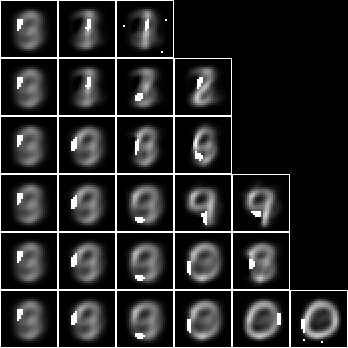Both the averaged slice and the fud underlying are shown in each image to indicate where the attention is during decomposition.

### Model 35 - All pixels

Now consider a compiled inducer. NIST_model35.json is induced by NIST_engine35.py. The sample is the entire training set of 60,000 events.

NIST_engine35 may be run as follows (see README) -

python3 NIST_engine35.py >NIST_engine35.log 2>&1


The first section loads the sample,

    (uu,hr) = nistTrainBucketedIO(2)

digit = VarStr("digit")
vv = uvars(uu)
vvl = sset([digit])
vvk = vv - vvl


Then the parameters are defined,

    model = "NIST_model35"
(wmax,lmax,xmax,omax,bmax,mmax,umax,pmax,fmax,mult,seed) = (2**11, 8, 2**10, 30, (30*3), 3, 2**8, 1, 127, 1, 5)


The engine runs in Python 3.5 64-bit on a Ubuntu 16.04 Intel(R) Xeon(R) Platinum 8175M CPU @ 2.50GHz using 2623MB in 24991 seconds.

Then the decomper is run,

    (uu1,df1) = decomperIO(uu,vvk,hr,wmax,lmax,xmax,omax,bmax,mmax,umax,pmax,fmax,mult,seed)


The decomper is defined in NISTDev,

def decomperIO(uu,vv,hr,wmax,lmax,xmax,omax,bmax,mmax,umax,pmax,fmax,mult,seed):


Then the model is written to NIST_model35.json,

    open(model+".json","w").write(decompFudsPersistentsEncode(decompFudsPersistent(df1)))


The summed alignment and the summed alignment valency-density are calculated,

    (a,ad) = summation(mult,seed,uu1,df1,hr1)
print("alignment: %.2f" % a)


The statistics are,

model cardinality: 4040
train size: 7500
alignment: 132688.71
alignment density: 64806.34


Finally the images are written out,

    pp = treesPaths(hrmult(uu1,df1,hr1))
bmwrite(model+".bmp",ppbm2(uu,vvk,28,1,2,pp))
bmwrite(model+"_1.bmp",ppbm(uu,vvk,28,1,2,pp))
bmwrite(model+"_2.bmp",ppbm(uu,vvk,28,2,2,pp))


where the bitmap helper functions are defined in NISTDev,

def ppbm(uu,vvk,b,c,d,pp):
kk = []
for ll in pp:
jj = []
for ((_,ff),hrs) in ll:
jj.append(bmborder(1,bmmax(hrbm(b,c,d,hrhrred(hrs,vvk)),0,0,hrbm(b,c,d,qqhr(d,uu,vvk,fund(ff))))))
kk.append(bminsert(bmempty((b*c)+2,((b*c)+2)*max([len(ii) for ii in pp])),0,0,bmhstack(jj)))
return bmvstack(kk)

def ppbm2(uu,vvk,b,c,d,pp):
kk = []
for ll in pp:
jj = []
for ((_,ff),hrs) in ll:
jj.append(bmborder(1,hrbm(b,c,d,hrhrred(hrs,vvk))))
kk.append(bminsert(bmempty((b*c)+2,((b*c)+2)*max([len(ii) for ii in pp])),0,0,bmhstack(jj)))
return bmvstack(kk)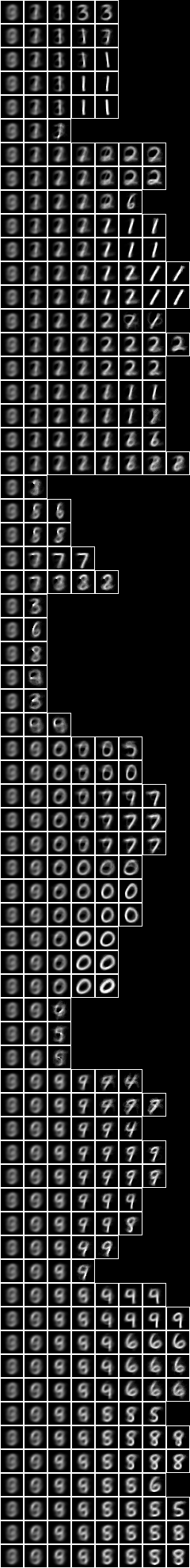Now with the fud underlying superimposed on the averaged slice,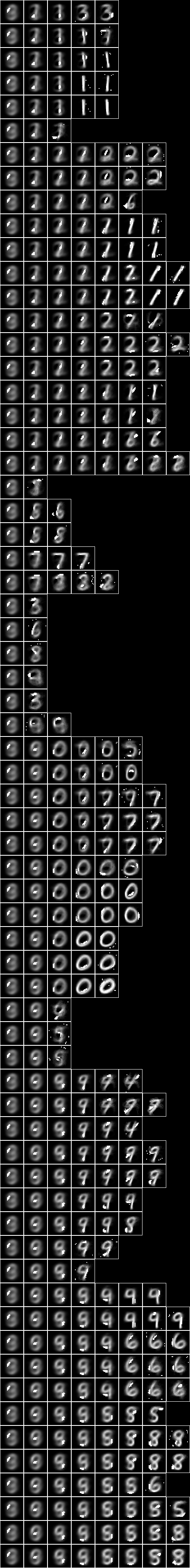### Model 34 - Averaged pixels

The pixel variables can be averaged as well as bucketed to reduce the dimension from 28x28 to 9x9. The NIST_model34.json is induced by NIST_engine34.py. The sample is the entire training set of 60,000 events. The first section loads the sample,

    (uu,hr) = nistTrainBucketedAveragedIO (8,9,0)


The parameters are defined,

    model = "NIST_model34"
(wmax,lmax,xmax,omax,bmax,mmax,umax,pmax,fmax,mult,seed) = (2**11, 8, 2**10, 30, (30*3), 3, 2**8, 1, 127, 1, 5)


The engine runs on a Python 3.5 64-bit on a Ubuntu 16.04 Intel(R) Xeon(R) Platinum 8175M CPU @ 2.50GHz using 2640MB in 13792 seconds.

The statistics are,

model cardinality: 4553
train size: 7500
alignment: 79321.16
alignment density: 37342.43


Imaging the fud decomposition,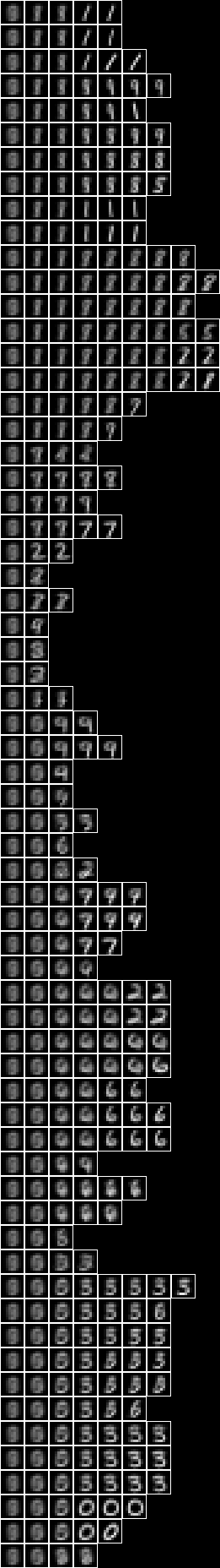Now with the fud underlying superimposed on the averaged slice,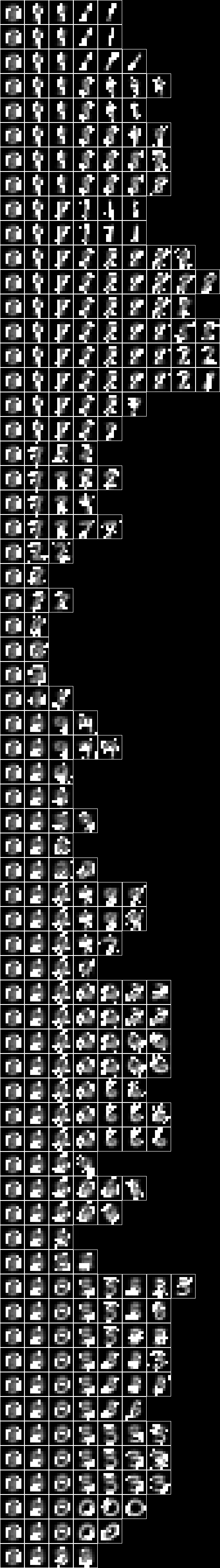### Model 5 - 15-fud square regions of 10x10 pixels

Now consider a 15-fud induced model applied to 7500 events of randomly chosen square regions of 10x10 pixels from the training sample. We shall run it in the interpreter,

from NISTDev import *

(uu,hrtr) = nistTrainBucketedRegionRandomIO(2,10,17)

digit = VarStr("digit")
vv = uvars(uu)
vvl = sset([digit])
vvk = vv - vvl

hr = hrev([i for i in range(hrsize(hrtr)) if i % 8 == 0],hrtr)

(wmax,lmax,xmax,omax,bmax,mmax,umax,pmax,fmax,mult,seed) = (2**10, 8, 2**10, 10, (10*3), 3, 2**8, 1, 15, 3, 5)

(uu1,df) = decomperIO(uu,vvk,hr,wmax,lmax,xmax,omax,bmax,mmax,umax,pmax,fmax,mult,seed)



This runs in the Python 3.7 32-bit interpreter on a Windows 7 Xeon CPU 5150 @ 2.66GHz in 5212 seconds.

summation(mult,seed,uu1,df,hr)
# (137784.2735394567, 68689.16514478576)

len(dfund(df))
# 98

len(fvars(dfff(df)))
# 582

open("NIST_model5.json","w").write(decompFudsPersistentsEncode(decompFudsPersistent(df)))



Imaging the fud decomposition,

pp = treesPaths(hrmult(uu1,df,hr))

rpln([[hrsize(hr) for (_,hr) in ll] for ll in pp])
# [7500, 3269, 1425, 785]
# [7500, 3269, 1796, 653]
# [7500, 3269, 1796, 1134]
# [7500, 3873, 1365, 924]
# [7500, 3873, 2495, 844]
# [7500, 3873, 2495, 1584, 1086, 736]

file = "NIST.bmp"

bmwrite(file,ppbm(uu,vvk,10,3,2,pp))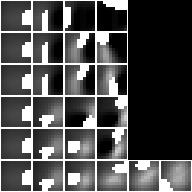Both the averaged slice and the fud underlying are shown in each image to indicate where the attention is during decomposition.

Now showing just the averaged slice,

bmwrite(file,ppbm2(uu,vvk,10,3,2,pp))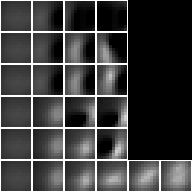### Model 6 - Square regions of 10x10 pixels

Now consider a compiled inducer. NIST_model6.json is induced by NIST_engine6.py. The sample is the entire training set of 60,000 events.

The first section loads the sample,

    (uu,hr) = nistTrainBucketedRegionRandomIO(2,10,17)


The parameters are defined,

    model = "NIST_model6"
(wmax,lmax,xmax,omax,bmax,mmax,umax,pmax,fmax,mult,seed) = (2**11, 8, 2**10, 30, (30*3), 3, 2**8, 1, 127, 1, 5)


The engine runs on a Python 3.5 64-bit on a Ubuntu 16.04 Intel(R) Xeon(R) Platinum 8175M CPU @ 2.50GHz using 1456MB in 20338 seconds.

The statistics are,

model cardinality: 3842
train size: 7500
alignment: 158224.09
alignment density: 78504.47


Imaging the fud decomposition,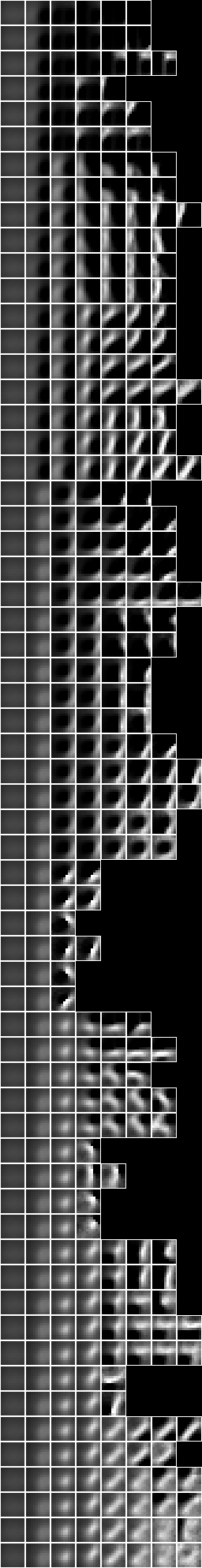Now with the fud underlying superimposed on the averaged slice,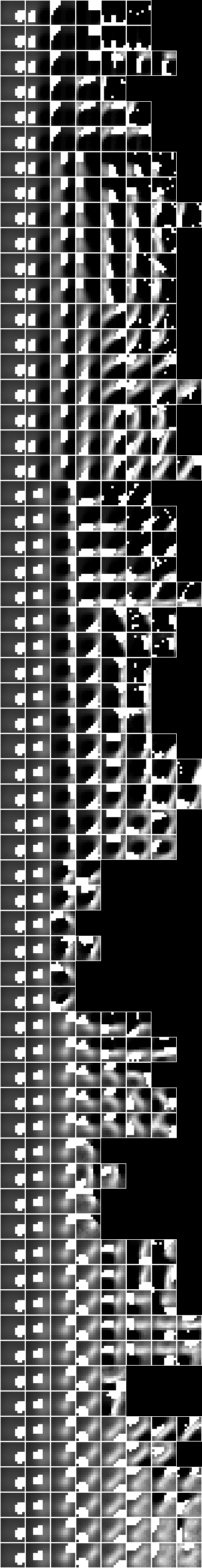### Model 21 - Square regions of 15x15 pixels

Now consider a compiled inducer. NIST_model21.json is induced by NIST_engine21.py. The sample is the entire training set of 60,000 events.

The first section loads the sample,

    (uu,hr) = nistTrainBucketedRegionRandomIO(2,15,17)


The parameters are defined,

    model = "NIST_model21"
(wmax,lmax,xmax,omax,bmax,mmax,umax,pmax,fmax,mult,seed) = (2**11, 8, 2**10, 30, (30*3), 3, 2**8, 1, 127, 1, 5)


The engine runs on a Python 3.5 64-bit on a Ubuntu 16.04 Intel(R) Xeon(R) Platinum 8175M CPU @ 2.50GHz using 1728 MB in 23497 seconds.

The statistics are,

model cardinality: 4082
train size: 7500
alignment: 195132.96
alignment density: 97210.93


Imaging the fud decomposition,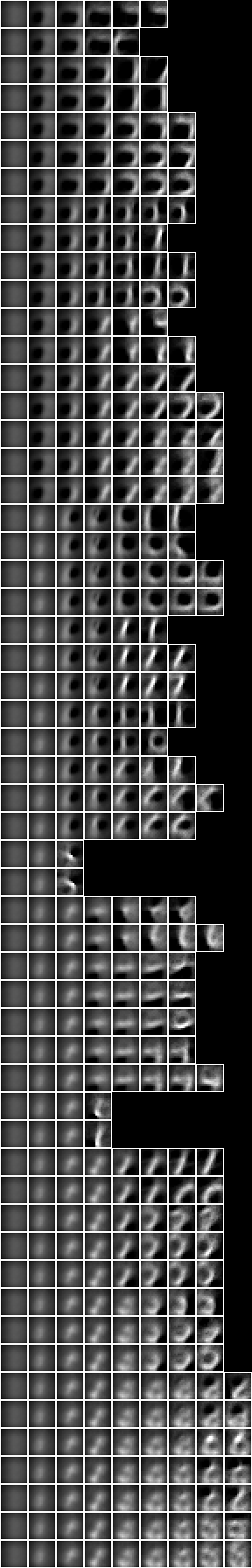Now with the fud underlying superimposed on the averaged slice,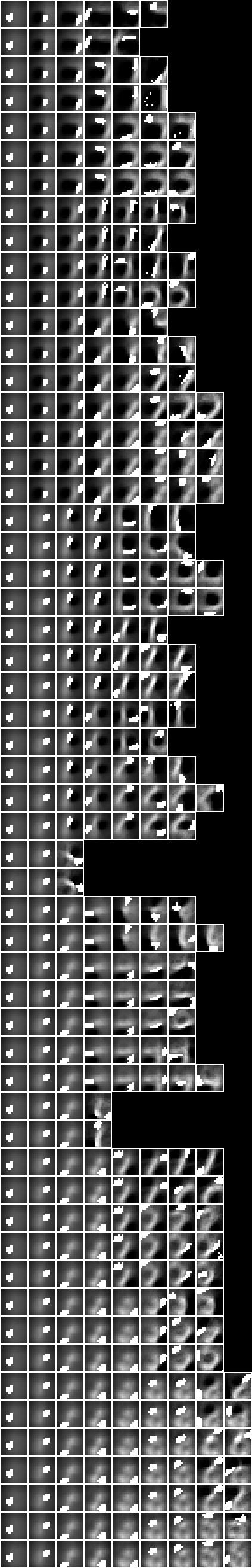### Model 10 - Centred square regions of 11x11 pixels

NIST_model10.json is induced by NIST_engine10.py. The sample is the entire training set of 60,000 events.

The first section loads the sample,

    (uu,hrtr) = nistTrainBucketedRegionRandomIO(2,11,17)

u = stringsVariable("<6,6>")
hr = hrhrsel(hrtr,aahr(uu,single(llss([(u,ValInt(1))]),1)))

digit = VarStr("digit")
vv = uvars(uu)
vvl = sset([digit])
vvk = vv - vvl


Then the parameters are defined,

    model = "NIST_model10"
(wmax,lmax,xmax,omax,bmax,mmax,umax,pmax,fmax,mult,seed) = (2**11, 8, 2**10, 30, (30*3), 3, 2**8, 1, 127, 1, 5)


The engine runs on a Python 3.5 64-bit on a Ubuntu 16.04 Intel(R) Xeon(R) Platinum 8175M CPU @ 2.50GHz using 1094 MB in 17678 seconds.

The statistics are,

model cardinality: 3711
train size: 2311
alignment: 53897.90
alignment density: 26846.93


Imaging the fud decomposition,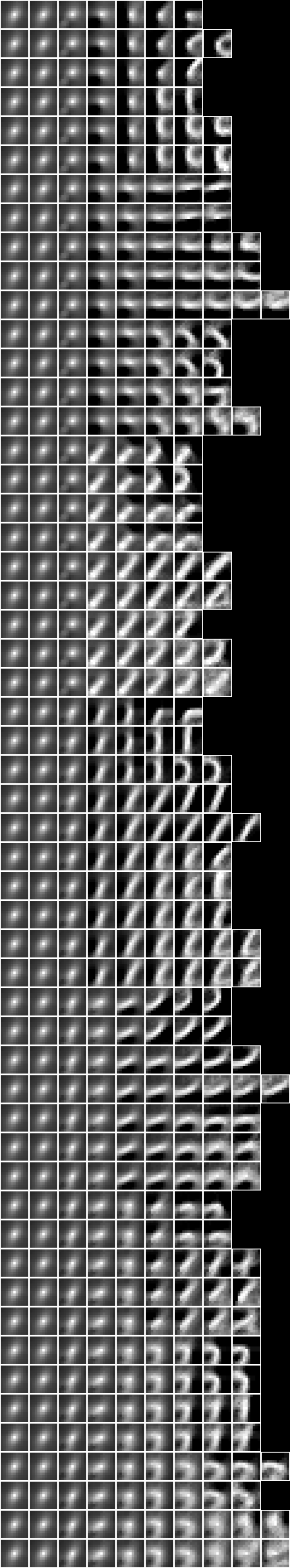Now with the fud underlying superimposed on the averaged slice,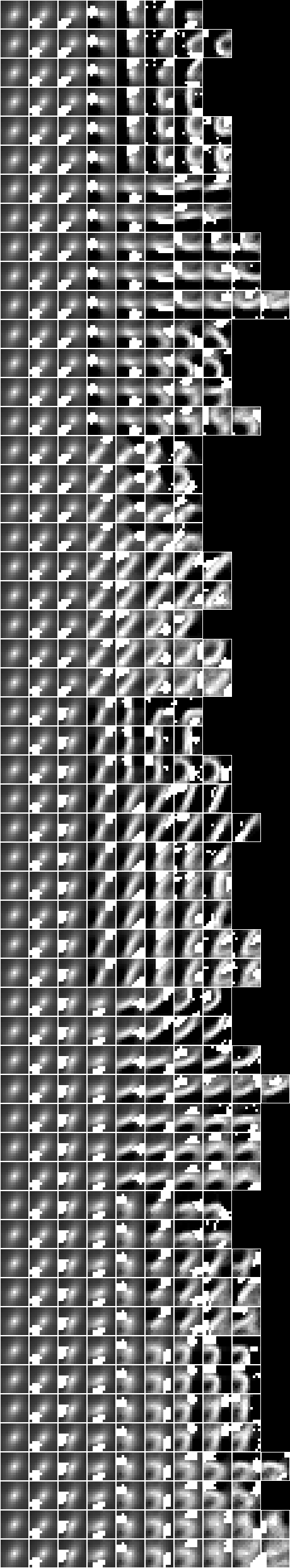### Model 24 - Two level over 10x10 regions

Now consider 5x5 copies of the Model 6 - Square regions of 10x10 pixels over the whole query substrate. NIST_model24.json is induced by NIST_engine24.py. The sample is the entire training set of 60,000 events.

The first section loads the underlying sample,

    (uu,hr) = nistTrainBucketedRegionRandomIO(2,10,17)


Then the underlying decomposition is loaded, reduced, converted to a nullable fud, and reframed at level 1,

    df1 = dfIO('./NIST_model6.json')

uu1 = uunion(uu,fsys(dfff(df1)))

ff1 = fframe(refr1(1),dfnul(uu1,dfred(uu1,df1,hr),1))


where

def refr1(k):
def refr1_f(v):
if isinstance(v, VarPair):
(w,i) = v._rep
if isinstance(w, VarPair):
(f,l) = w._rep
if isinstance(f, VarInt):
return VarPair((VarPair((VarPair((VarPair((VarInt(k),f)),VarInt(0))),l)),i))
elif isinstance(f, VarPair):
(f1,g) = f._rep
return VarPair((VarPair((VarPair((VarPair((VarInt(k),f1)),g)),l)),i))
return v
return refr1_f


    (uu,hr) = nistTrainBucketedIO(2)

digit = VarStr("digit")
vv = uvars(uu)
vvl = sset([digit])
vvk = vv - vvl


Then the underlying model is copied and reframed at level 2,

    gg1 = sset()
for x in [2,6,10,14,18]:
for y in [2,6,10,14,18]:
gg1 |= fframe(refr2(x,y),ff1)

uu1 = uunion(uu,fsys(gg1))


where

def refr2(x,y):
def refr2_f(v):
if isinstance(v, VarPair) and isinstance(v._rep, VarInt) and isinstance(v._rep, VarInt):
(i,j) = v._rep
return VarPair((VarInt((x-1)+i._rep),VarInt((y-1)+j._rep)))
return VarPair((v,VarStr("(" + str(x) + ";" + str(y) + ")")))
return refr2_f


Then the parameters are defined,

    model = "NIST_model24"
(wmax,lmax,xmax,omax,bmax,mmax,umax,pmax,fmax,mult,seed) = (2**11, 8, 2**10, 30, (30*3), 3, 2**8, 1, 127, 1, 5)


Then the decomper is run,

    (uu2,df2) = decomperIO(uu1,gg1,hr,wmax,lmax,xmax,omax,bmax,mmax,umax,pmax,fmax,mult,seed)


where

def decomperIO(uu,ff,hr,wmax,lmax,xmax,omax,bmax,mmax,umax,pmax,fmax,mult,seed):


The engine runs on a Python 3.5 64-bit on a Ubuntu 16.04 Intel(R) Xeon(R) Platinum 8175M CPU @ 2.50GHz using 12663 MB in 67664 seconds.

The statistics are,

model cardinality: 5966
train size: 7500
alignment: 190022.26
alignment density: 89892.41


Imaging the fud decomposition,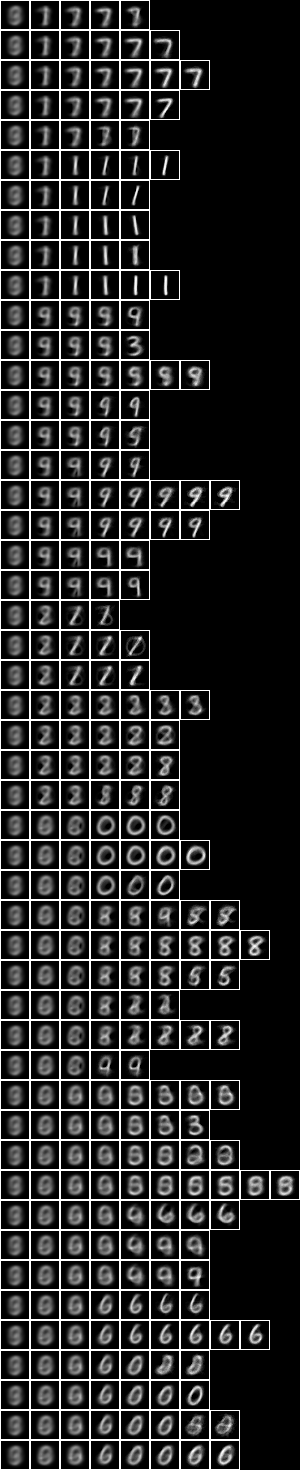Now with the fud underlying superimposed on the averaged slice,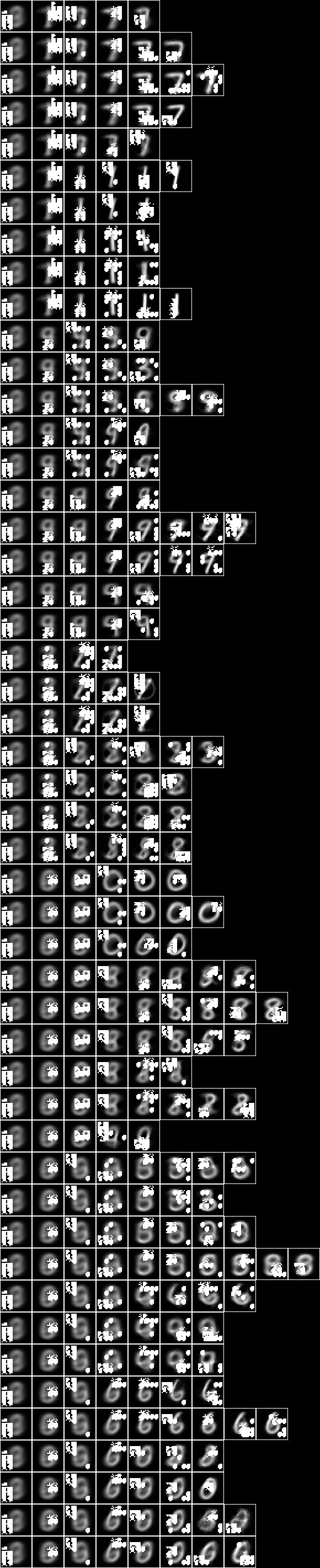### Model 25 - Two level over 15x15 regions

Now consider 5x5 copies of the Model 21 - Square regions of 15x15 pixels over the whole query substrate. NIST_model25.json is induced by NIST_engine25.py. The sample is the entire training set of 60,000 events.

The engine is very similar to Model 24 - Two level over 10x10 regions. The first section loads the underlying sample,

    (uu,hr) = nistTrainBucketedRegionRandomIO(2,15,17)


Then the underlying decomposition is loaded, reduced, converted to a nullable fud, and reframed at level 1,

    df1 = dfIO('./NIST_model21.json')

uu1 = uunion(uu,fsys(dfff(df1)))

ff1 = fframe(refr1(1),dfnul(uu1,dfred(uu1,df1,hr),1))


    (uu,hr) = nistTrainBucketedIO(2)

digit = VarStr("digit")
vv = uvars(uu)
vvl = sset([digit])
vvk = vv - vvl


Then the underlying model is copied and reframed at level 2,

    gg1 = sset()
for x in [1,4,7,10,13]:
for y in [1,4,7,10,13]:
gg1 |= fframe(refr2(x,y),ff1)

uu1 = uunion(uu,fsys(gg1))


Then the parameters are defined,

    model = "NIST_model25"
(wmax,lmax,xmax,omax,bmax,mmax,umax,pmax,fmax,mult,seed) = (2**11, 8, 2**10, 30, (30*3), 3, 2**8, 1, 127, 1, 5)


Then the decomper is run,

    (uu2,df2) = decomperIO(uu1,gg1,hr,wmax,lmax,xmax,omax,bmax,mmax,umax,pmax,fmax,mult,seed)


The engine runs on a Python 3.5 64-bit on a Ubuntu 16.04 Intel(R) Xeon(R) Platinum 8175M CPU @ 2.50GHz using 12349MB in 52735 seconds.

The statistics are,

model cardinality: 5787
train size: 7500
alignment: 174749.41
alignment density: 78155.56


Imaging the fud decomposition,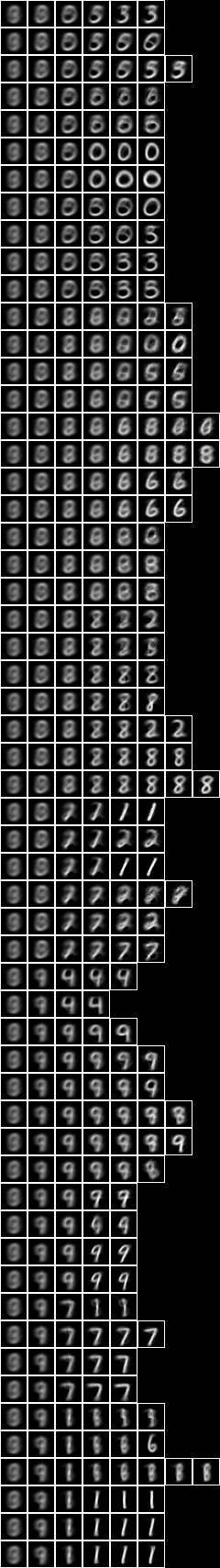Now with the fud underlying superimposed on the averaged slice,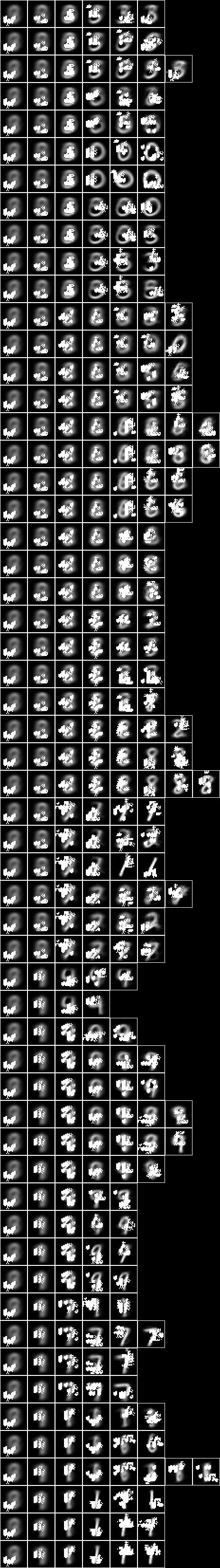### Model 26 - Two level over centred square regions of 11x11 pixels

Now consider 5x5 copies of the Model 10 - Centred square regions of 11x11 pixels over the whole query substrate. NIST_model26.json is induced by NIST_engine26.py. The sample is the entire training set of 60,000 events.

The engine is very similar to Model 24 - Two level over 10x10 regions and Model 25 - Two level over 15x15 regions. The first section loads the underlying sample,

    (uu,hr) = nistTrainBucketedRegionRandomIO(2,11,17)


Then the underlying decomposition is loaded, reduced, converted to a nullable fud, and reframed at level 1,

    df1 = dfIO('./NIST_model10.json')

uu1 = uunion(uu,fsys(dfff(df1)))

ff1 = fframe(refr1(1),dfnul(uu1,dfred(uu1,df1,hr),1))


    (uu,hr) = nistTrainBucketedIO(2)

digit = VarStr("digit")
vv = uvars(uu)
vvl = sset([digit])
vvk = vv - vvl


Then the underlying model is copied and reframed at level 2,

    gg1 = sset()
for x in [1,4,7,10,13]:
for y in [1,4,7,10,13]:
gg1 |= fframe(refr2(x,y),ff1)

uu1 = uunion(uu,fsys(gg1))


Then the parameters are defined,

    model = "NIST_model26"
(wmax,lmax,xmax,omax,bmax,mmax,umax,pmax,fmax,mult,seed) = (2**11, 8, 2**10, 30, (30*3), 3, 2**8, 1, 127, 1, 5)


Then the decomper is run,

    (uu2,df2) = decomperIO(uu1,gg1,hr,wmax,lmax,xmax,omax,bmax,mmax,umax,pmax,fmax,mult,seed)


The engine runs in Python 3.5 64-bit on a Ubuntu 16.04 Intel(R) Xeon(R) Platinum 8175M CPU @ 2.50GHz using 9280MB in 56259 seconds.

The statistics are,

model cardinality: 5671
train size: 7500
alignment: 206488.07
alignment density: 98883.55


Imaging the fud decomposition,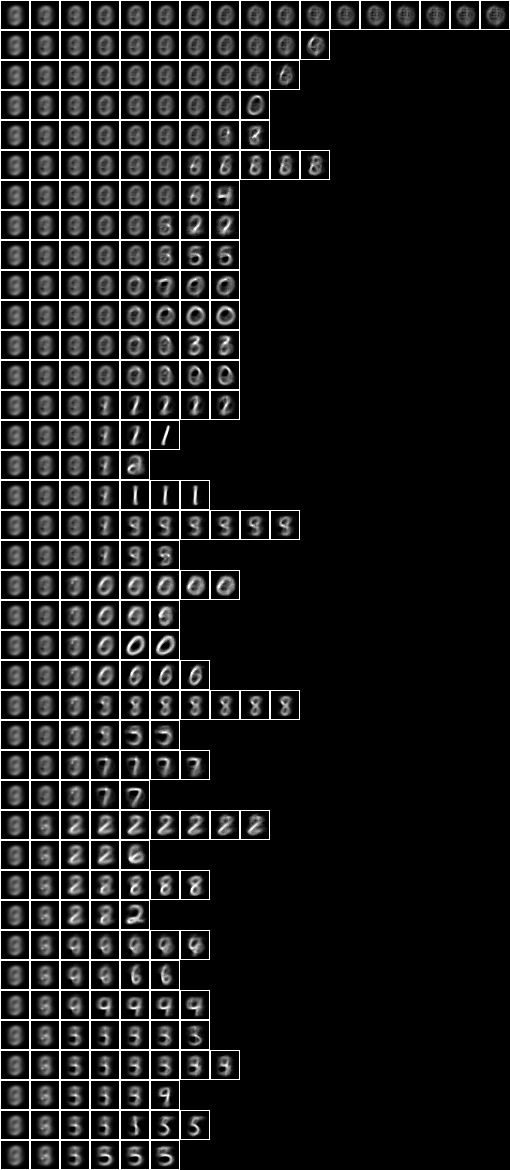Now with the fud underlying superimposed on the averaged slice,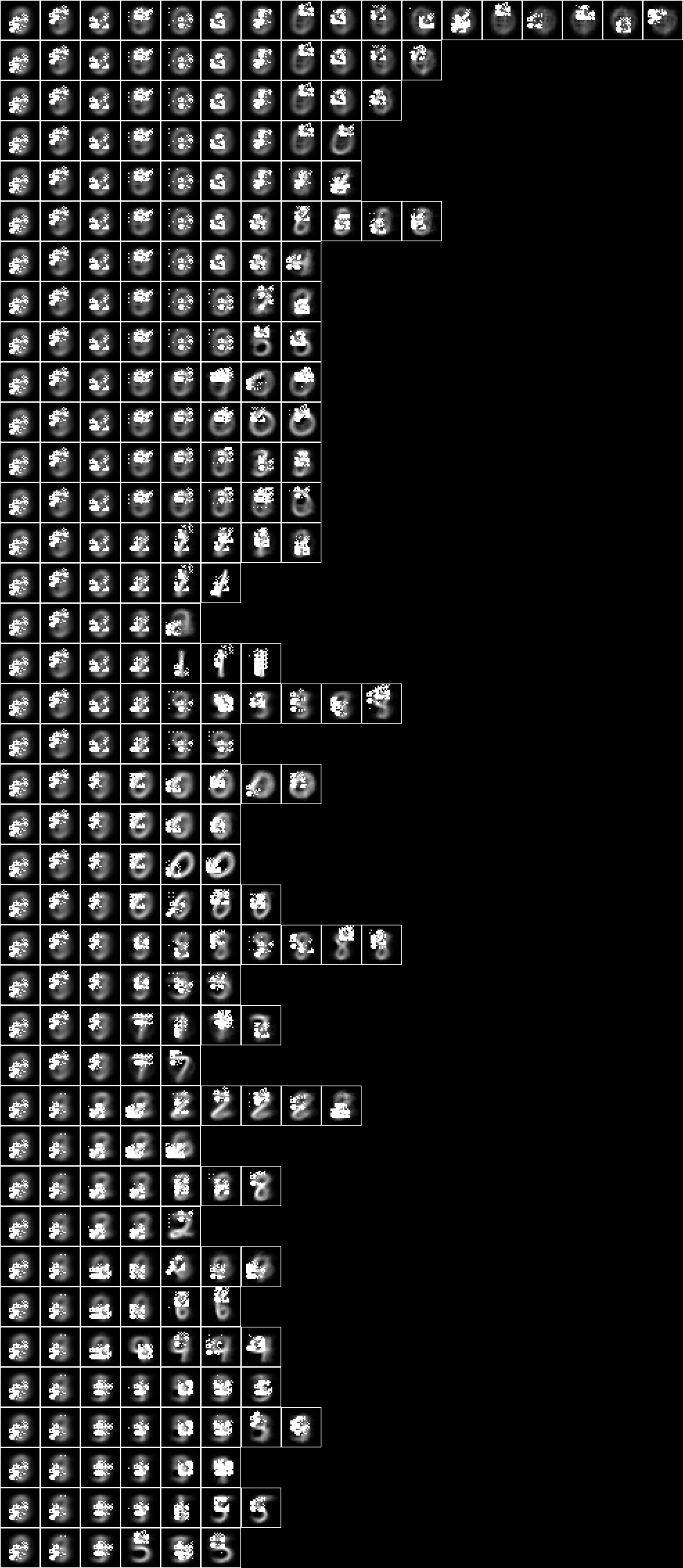### NIST test

The NIST_test.py is executed as follows,

python3 NIST_test.py NIST_model2

model: NIST_model2
train size: 7500
model cardinality: 515
nullable fud cardinality: 688
nullable fud derived cardinality: 147
nullable fud underlying cardinality: 134
ff label ent: 1.4174827406154242
test size: 1000
effective size: 988
matches: 436


This runs on model 2 in a Python 3.5 64-bit interpreter on a Ubuntu 16.04 Intel(R) Xeon(R) Platinum 8175M CPU @ 2.50GHz in 270 seconds.

The first section loads the sample and the model,

    model = argv
(uu,hrtr) = nistTrainBucketedIO(2)
hr = hrev([i for i in range(hrsize(hrtr)) if i % 8 == 0],hrtr)
digit = VarStr("digit")
vv = uvars(uu)
vvl = sset([digit])
vvk = vv - vvl
df1 = dfIO(model + '.json')


Then the fud decomposition fud is created,

    uu1 = uunion(uu,fsys(dfff(df1)))
ff1 = dfnul(uu1,df1,9)


Then the label entropy is calculated,

    uu1 = uunion(uu,fsys(ff1))
hr1 = hrfmul(uu1,ff1,hr)
print("ff label ent: %.16f" % hrlent(uu1,hr1,fder(ff1),vvl))


Lastly, the test history is loaded and the query effectiveness and query accuracy is calculated,

    (uu,hrte) = nistTestBucketedIO(2)
hrq = hrev([i for i in range(hrsize(hrte)) if i % 10 == 0],hrte)
hrq1 = hrfmul(uu1,ff1,hrq)
print("effective size: %d" % int(size(mul(hhaa(hrhh(uu1,hrhrred(hrq1,fder(ff1)))),eff(hhaa(hrhh(uu1,hrhrred(hr1,fder(ff1)))))))))
print("matches: %d" % len([rr for (_,ss) in hhll(hrhh(uu1,hrhrred(hrq1,fder(ff1)|vvl))) for qq in [single(ss,1)] for rr in [araa(uu1,hrred(hrhrsel(hr1,hhhr(uu1,aahh(red(qq,fder(ff1))))),vvl))] if size(rr) > 0 and size(mul(amax(rr),red(qq,vvl))) > 0]))


### NIST conditional

Given an induced model, this engine finds a semi-supervised submodel that predicts the label variables, $V_{\mathrm{l}}$, or digit, by optimising conditional entropy. The NIST_engine_cond.py is defined as follows.

The first section loads the parameters, sample and the model,

    valency = int(argv)
modelin = argv
kmax = int(argv)
omax = int(argv)
fmax = int(argv)
model = argv
(uu,hr) = nistTrainBucketedIO(valency)
digit = VarStr("digit")
vv = uvars(uu)
vvl = sset([digit])
vvk = vv - vvl
df1 = dfIO(modelin + '.json')


Then the fud decomposition fud is created,

    uu1 = uunion(uu,fsys(dfff(df1)))
ff1 = fframe(refr1(3),dfnul(uu1,df1,1))


Then the model is applied,

    uu1 = uunion(uu,fsys(ff1))
hr1 = hrfmul(uu1,ff1,hr)


Then the conditional entropy fud decomper is run,

    (uu2,df2) = decompercondrr(vvl,uu1,hr1,kmax,omax,fmax)
df21 = zzdf(funcsTreesMap(lambda xx:(xx,fdep(xx|ff1,fder(xx))),dfzz(df2)))


where

def decompercondrr(ll,uu,aa,kmax,omax,fmax):


Then the model is written to NIST_model36.json,

    open(model+".json","w").write(decompFudsPersistentsEncode(decompFudsPersistent(df21)))


Finally the images are written out,

    pp = treesPaths(hrmult(uu2,df21,hr1))
bmwrite(model+".bmp",ppbm2(uu,vvk,28,1,2,pp))
bmwrite(model+"_1.bmp",ppbm(uu,vvk,28,1,2,pp))
bmwrite(model+"_2.bmp",ppbm(uu,vvk,28,2,2,pp))


### Model 36 - Conditional level over 15-fud

Now run the conditional entropy fud decomper to create Model 36, the conditional level over 15-fud induced model of all pixels, Model 2,

python3 NIST_engine_cond.py 2 NIST_model2 1 5 15 NIST_model36 >NIST_engine36.log


This runs on on a Ubuntu 16.04 Pentium CPU G2030 @ 3.00GHz in 1883 MB memory in 231 seconds.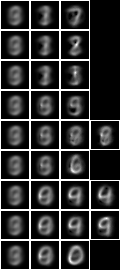Now with the fud underlying superimposed on the averaged slice,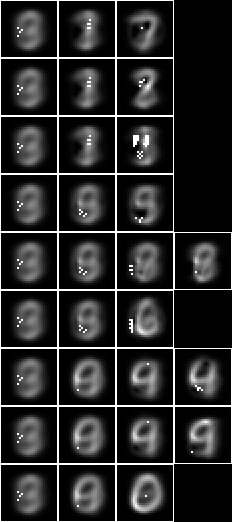If we run NIST test on the new model,

python3 NIST_test.py NIST_model36 >NIST_test_model36.log


we obtain the following statistics,

model: NIST_model36
train size: 7500
model cardinality: 162
nullable fud cardinality: 202
nullable fud derived cardinality: 15
nullable fud underlying cardinality: 63
ff label ent: 1.4004486362258195
test size: 1000
effective size: 1000
matches: 507


### Model 37 - Conditional level over all pixels

Now let us repeat the analysis of Model 36, but for the conditional level over 127-fud induced model of all pixels, Model 35. Model 37 -

python3 NIST_engine_cond.py 2 NIST_model35 1 5 127 NIST_model37 >NIST_engine37.log


The engine runs on a Python 3.5 64-bit on a Ubuntu 16.04 Intel(R) Xeon(R) Platinum 8175M CPU @ 2.50GHz using 12462 MB in 5497 seconds.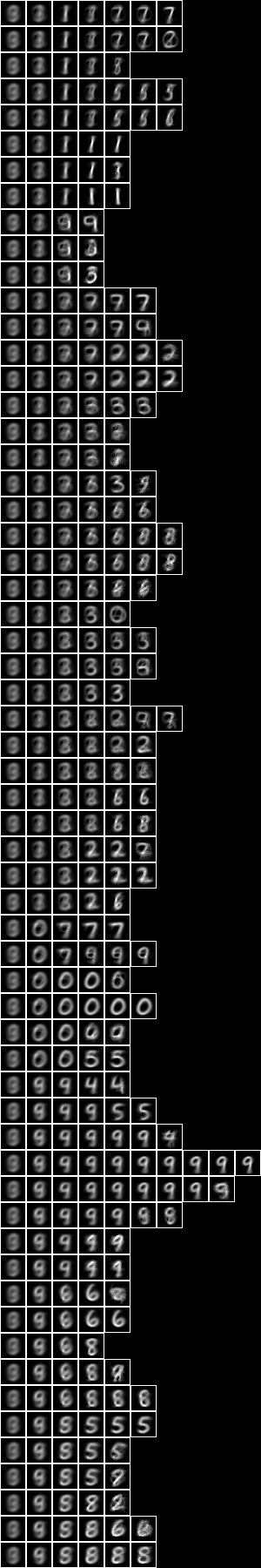Now with the fud underlying superimposed on the averaged slice,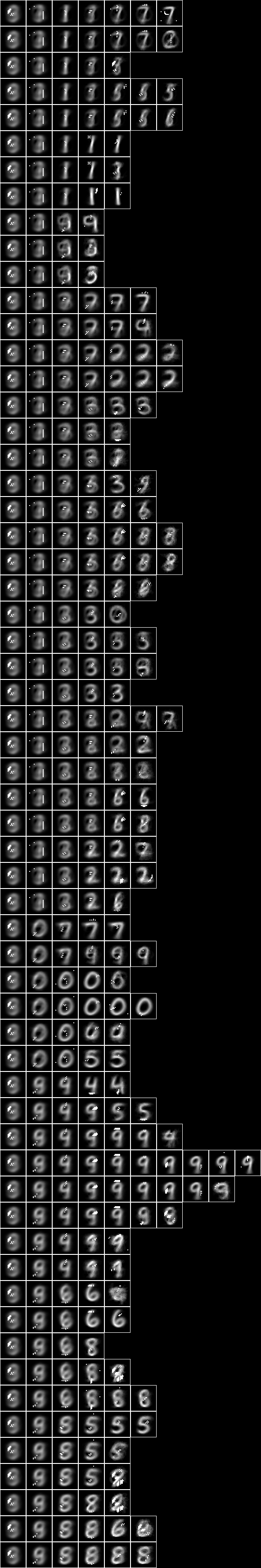If we run NIST test on the new model,

python3 NIST_test.py NIST_model37 >NIST_test_model37.log


we obtain the following statistics,

model: NIST_model37
train size: 7500
model cardinality: 663
nullable fud cardinality: 1039
nullable fud derived cardinality: 127
nullable fud underlying cardinality: 253
ff label ent: 0.6564998305260028
test size: 1000
effective size: 999
matches: 773


Repeating the same, but with 2-tuple conditional,

python3 NIST_engine_cond.py 2 NIST_model35 2 5 127 NIST_model39 >NIST_engine39.log


The engine runs on a Python 3.5 64-bit on a Ubuntu 16.04 Intel(R) Xeon(R) Platinum 8175M CPU @ 2.50GHz using 9281 MB in 10614 seconds.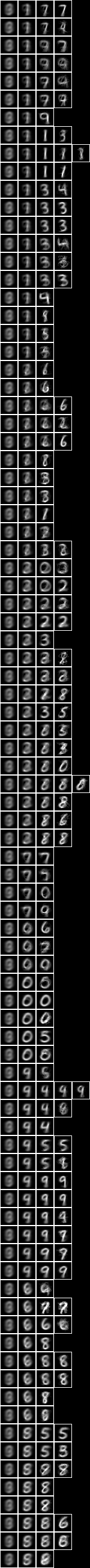Now with the fud underlying superimposed on the averaged slice,If we run NIST test on the new model,

python3 NIST_test.py NIST_model39 >NIST_test_model39.log


we obtain the following statistics,

model: NIST_model39
train size: 7500
model cardinality: 872
nullable fud cardinality: 1242
nullable fud derived cardinality: 127
nullable fud underlying cardinality: 301
ff label ent: 0.4036616947586324
test size: 1000
effective size: 979
matches: 797


### Model 38 - Conditional level over averaged pixels

Now let us repeat the analysis for the conditional level over 127-fud induced model of averaged pixels, Model 34.

The NIST_engine_cond_averaged.py is defined as follows. The first section loads the parameters, sample and the model,

    valency = int(argv)
offset = int(argv)
modelin = argv
kmax = int(argv)
omax = int(argv)
fmax = int(argv)
model = argv
digit = VarStr("digit")
vv = uvars(uu)
vvl = sset([digit])
vvk = vv - vvl
df1 = dfIO(modelin + '.json')


The remainder is as for Model 36.

Now run Model 38 -

python3 NIST_engine_cond_averaged.py 8 9 0 NIST_model34 1 5 127 NIST_model38 >NIST_engine38.log


The engine runs on a Python 3.5 64-bit on a Ubuntu 16.04 Intel(R) Xeon(R) Platinum 8175M CPU @ 2.50GHz using 10847 MB in 5517 seconds.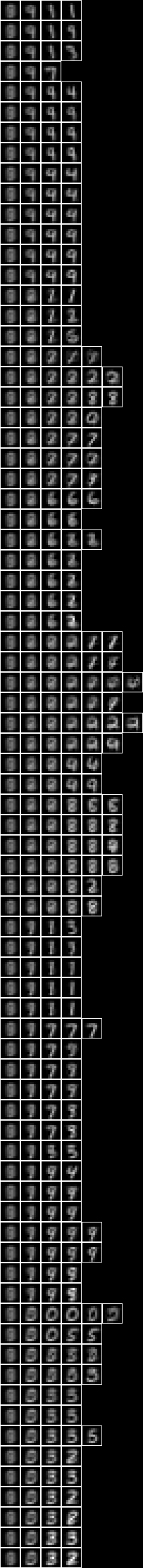Now with the fud underlying superimposed on the averaged slice,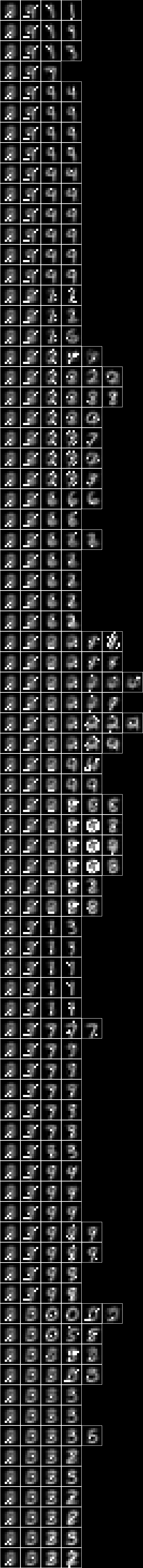### NIST test averaged

We must modify NIST test to allow for an averaged substrate. The NIST_test_averaged.py is executed as follows,

python3 NIST_test_averaged.py 8 9 0 NIST_model38 >NIST_test_model38.log


we obtain the following statistics,

model: NIST_model38
selected train size: 7500
model cardinality: 308
nullable fud cardinality: 683
nullable fud derived cardinality: 127
nullable fud underlying cardinality: 45
ff label ent: 0.6309812112866986
test size: 1000
effective size: 986
matches: 718


### NIST conditional over square regions

We can modify NIST conditional to copy an array of region models. The NIST_engine_cond_regions.py is defined follows, The first section loads the parameters, regional sample and the regional model,

    valency = int(argv)
seed = int(argv)
ufmax = int(argv)
locations = map(int, argv.split())
modelin = argv
kmax = int(argv)
omax = int(argv)
fmax = int(argv)
model = argv
df1 = dfIO(modelin + '.json')


Then the fud decomposition fud is created,

    uu1 = uunion(uu,fsys(dfff(df1)))
ff1 = fframe(refr1(3),dfnul(uu1,dflt(df1,ufmax),3)))


    (uu,hr) = nistTrainBucketedIO(valency)
digit = VarStr("digit")
vv = uvars(uu)
vvl = sset([digit])
vvk = vv - vvl


Then the model is copied,

    gg1 = sset()
for x in locations:
for y in locations:
gg1 |= fframe(refr2(x,y),ff1)


Then the model is applied,

    uu1 = uunion(uu,fsys(gg1))
hr1 = hrfmul(uu1,gg1,hr)


Then the conditional entropy fud decomper is run,

    (uu2,df2) = decompercondrr(vvl,uu1,hr1,kmax,omax,fmax)
df21 = zzdf(funcsTreesMap(lambda xx:(xx,fdep(xx|gg1,fder(xx))),dfzz(df2)))


where

def decompercondrr(ll,uu,aa,kmax,omax,fmax):


The remainder of the engine is as for NIST conditional.

### Model 43 - Conditional level over square regions of 15x15 pixels

Now run the regional conditional entropy fud decomper to create Model 43, the conditional level over a 7x7 array of 127-fud induced models of square regions of 15x15 pixels, Model 21,

python3 NIST_engine_cond_regions.py 2 15 17 31 "1 3 5 7 9 11 13" NIST_model21 1 5 127 NIST_model43 >NIST_engine43.log


The engine runs on a Python 3.5 64-bit on a Ubuntu 16.04 Intel(R) Xeon(R) Platinum 8175M CPU @ 2.50GHz using 1546 MB in 7129 seconds.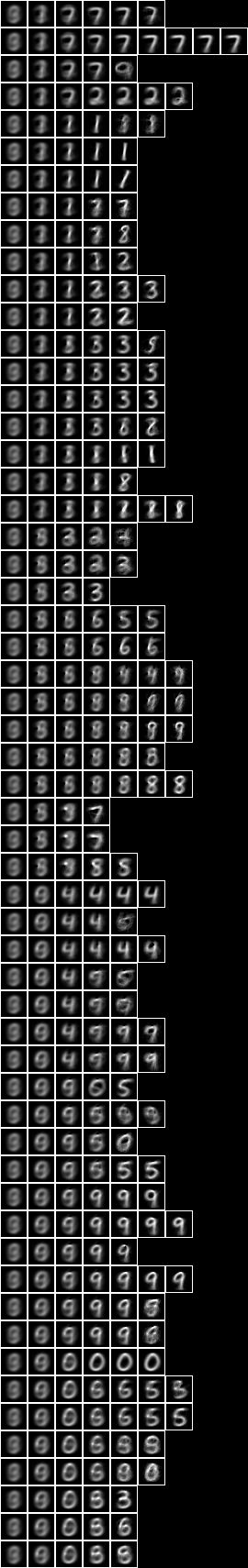Now with the fud underlying superimposed on the averaged slice,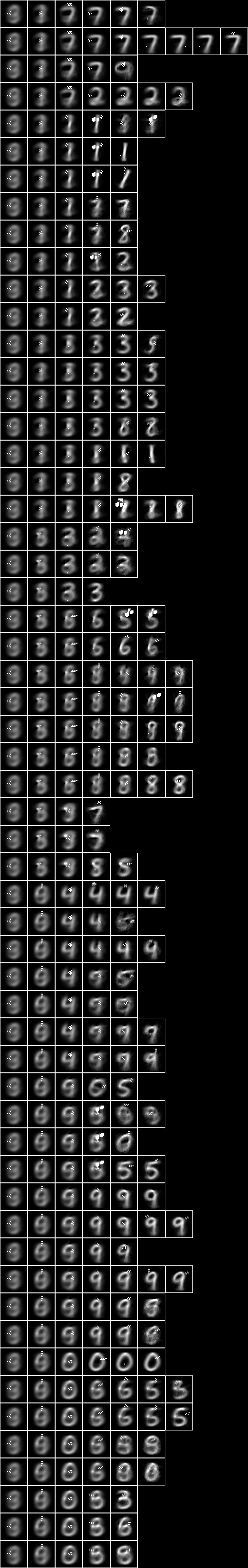If we run NIST test on the new model,

python3 NIST_test.py NIST_model43 >NIST_test_model43.log


we obtain the following statistics,

model: NIST_model43
train size: 7500
model cardinality: 868
nullable fud cardinality: 1244
nullable fud derived cardinality: 127
nullable fud underlying cardinality: 218
ff label ent: 0.6758031753055889
test size: 1000
effective size: 999
matches: 770


### Model 40 - Conditional level over two level over 10x10 regions

Now run the conditional entropy fud decomper over the 2-level induced model Model 24 to create Model 40,

python3 NIST_engine_cond.py 2 NIST_model24 1 5 127 NIST_model40 >NIST_engine40.log


The engine runs on a Python 3.5 64-bit on a Ubuntu 16.04 Intel(R) Xeon(R) Platinum 8175M CPU @ 2.50GHz using 17084 MB in 33159 seconds.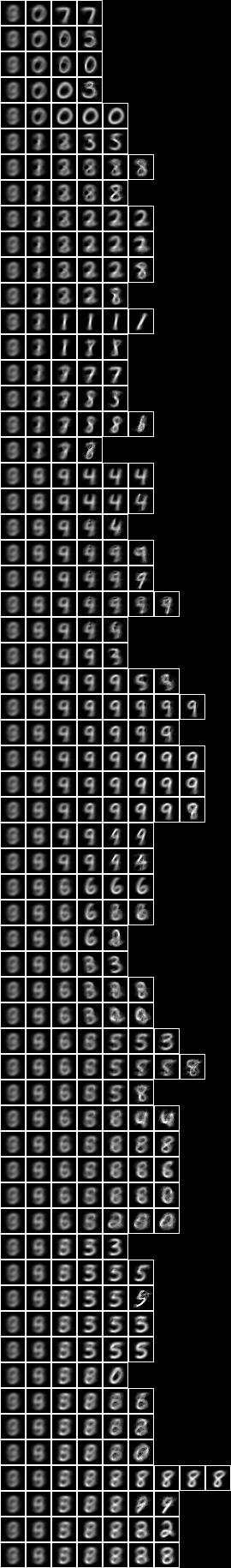Now with the fud underlying superimposed on the averaged slice,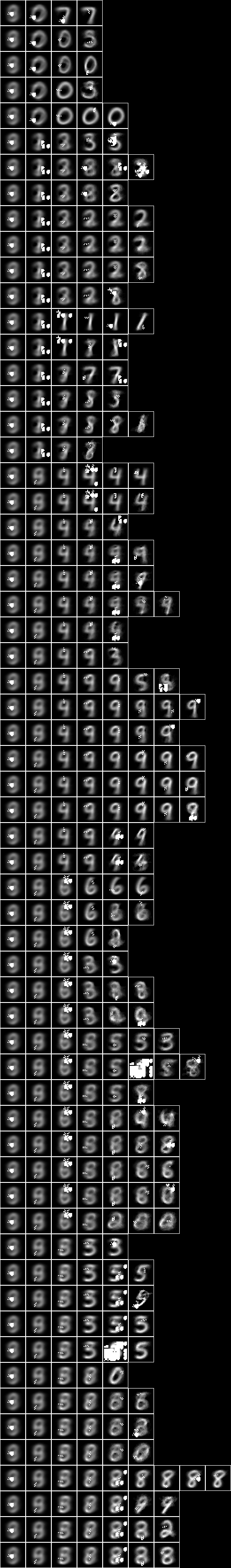If we run NIST test on the new model,

python3 NIST_test.py NIST_model40 >NIST_test_model40.log


we obtain the following statistics,

model: NIST_model40
train size: 7500
model cardinality: 2140
nullable fud cardinality: 2516
nullable fud derived cardinality: 127
nullable fud underlying cardinality: 562
ff label ent: 0.5836689910897102
test size: 1000
effective size: 997
matches: 802


### Model 41 - Conditional level over two level over 15x15 regions

Now run the conditional entropy fud decomper over the 2-level induced model Model 25 to create Model 41,

python3 NIST_engine_cond.py 2 NIST_model25 1 5 127 NIST_model41 >NIST_engine41.log


The engine runs on a Python 3.5 64-bit on a Ubuntu 16.04 Intel(R) Xeon(R) Platinum 8175M CPU @ 2.50GHz using 14880 MB in 8067 seconds.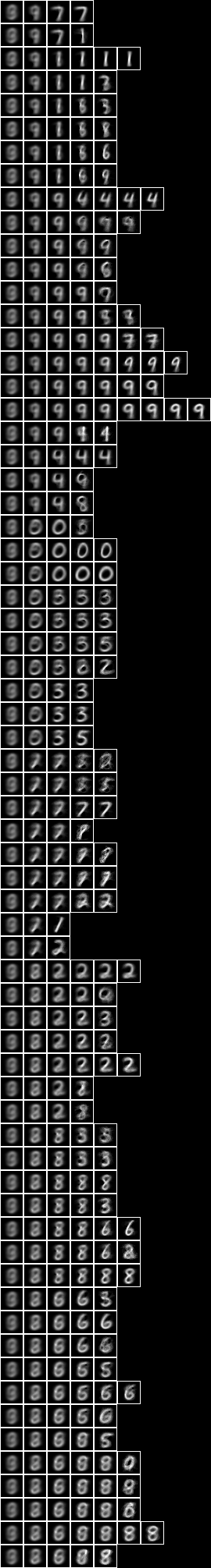Now with the fud underlying superimposed on the averaged slice,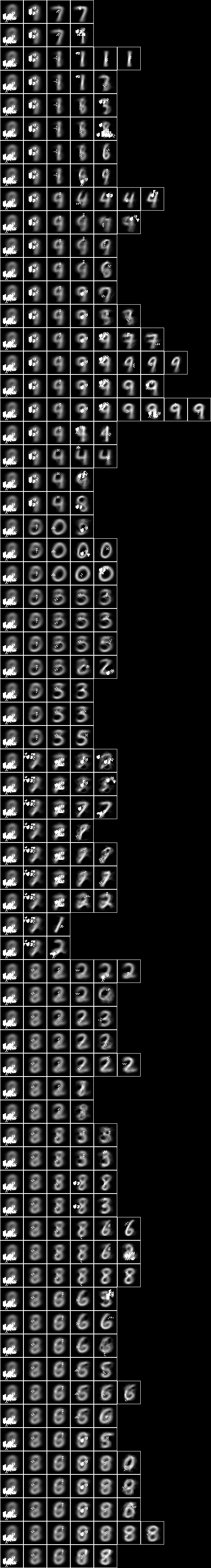If we run NIST test on the new model,

python3 NIST_test.py NIST_model41 >NIST_test_model41.log


we obtain the following statistics,

model: NIST_model41
train size: 7500
model cardinality: 1264
nullable fud cardinality: 1639
nullable fud derived cardinality: 127
nullable fud underlying cardinality: 421
ff label ent: 0.5874380803591093
test size: 1000
effective size: 998
matches: 778


### Model 42 - Conditional level over centred square regions of 11x11 pixels

Now run the conditional entropy fud decomper over the 2-level induced model Model 26 to create Model 42,

python3 NIST_engine_cond.py 2 NIST_model26 1 5 127 NIST_model42 >NIST_engine42.log


The engine runs on a Python 3.5 64-bit on a Ubuntu 16.04 Intel(R) Xeon(R) Platinum 8175M CPU @ 2.50GHz using 16430 MB in 10313 seconds.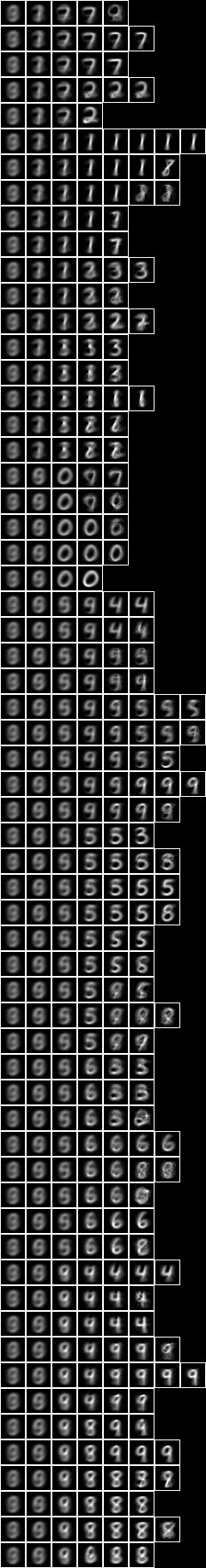Now with the fud underlying superimposed on the averaged slice,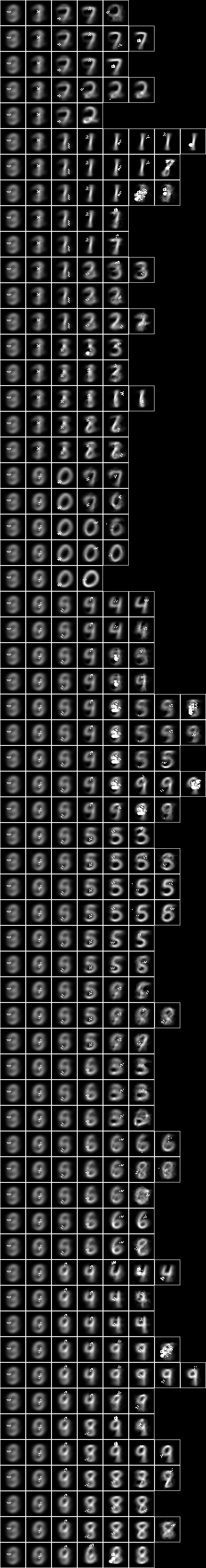If we run NIST test on the new model,

python3 NIST_test.py NIST_model42 >NIST_test_model42.log


we obtain the following statistics,

model: NIST_model42
train size: 7500
model cardinality: 772
nullable fud cardinality: 1149
nullable fud derived cardinality: 127
nullable fud underlying cardinality: 274
ff label ent: 0.6004072422056215
test size: 1000
effective size: 1000
matches: 785


top